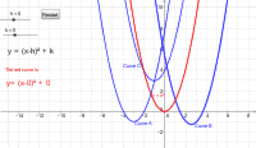Author:
Mr Creamer
Topic:
Equations
Quadratic curves and Equations The General Form: y = ax²+bx +c The Completed Square Form y = a(x-h)² +k The Factorised Form y= a(x-p)(x-q)
•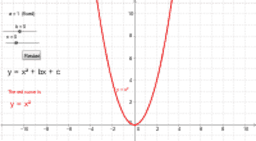### Quadratic curves and Equations Set 1

•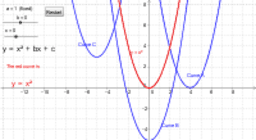### Quadratic curves and Equations Set 2

•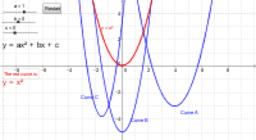### Quadratic curves and Equations Set 3

•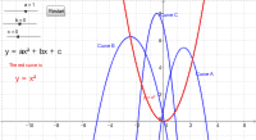### Quadratic curves and Equations Set 4

•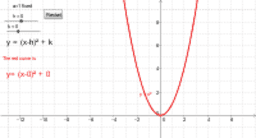### Quadratic curves and Equations Set 5 h&k

•